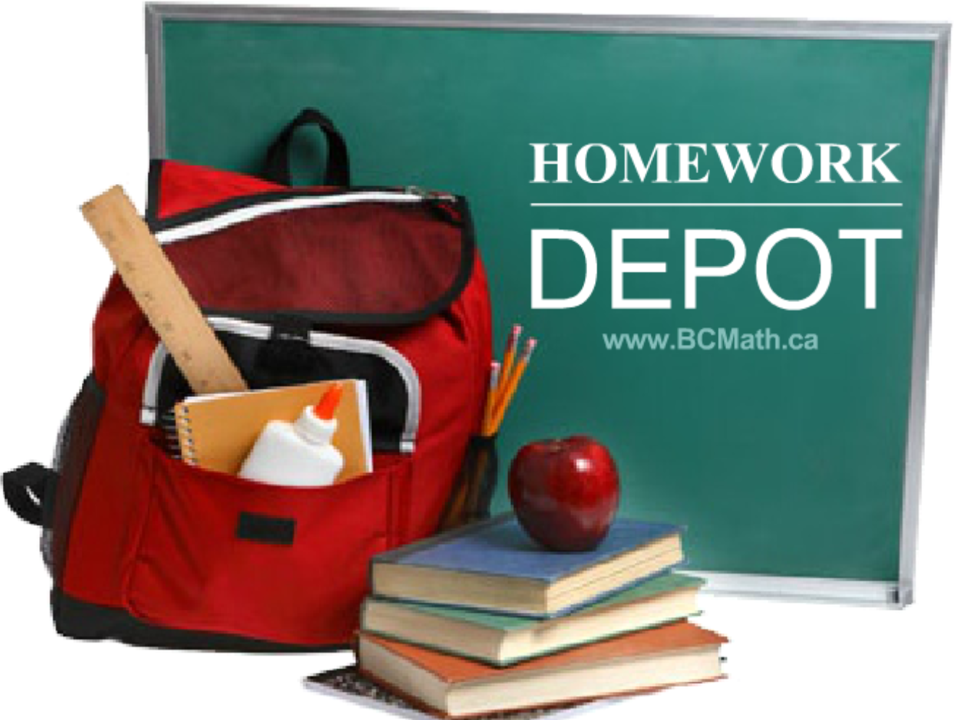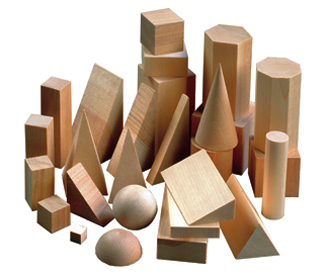Math 10 Honours Chapter 1:

•  CTS
•  1.1
•  1.2
•  1.3
•  1.4
•  1.5
•  1.6

PPT

•  CTS
•  1.1
•  1.2
•  1.3

•  1.4

•  1.5
•  1.5a
•  1.5b
•  1.6

•  Rev.

In this section, students will learn to:

• Convert Quadratic Functions from Standard to General Form
• Graphing Absolute value, Inverse and Reciprocal of Quadratic Functions
Click for HomeworkMath 10 Honours Chapter 2:

•  2.1

•  2.2
•  2.3
•  2.4
•  2.5

PPT

•  2.1

•  2.2
•  2.3
•  2.4

•  2.5

•  Rev.

Sequences and Series

In this section, students will learn to:

• Calculate the value of each term in a geometric and arithmetic sequence
• Evaluate the sum of a series
• Explore various types of Recursive sequences and series
• Evaluate Sigma Notations
Click for HomeworkMath 10 Honours Chapter 3:

•  3.1

•  3.2
•  3.3

•  3.4
•  3.5

•  3.6

•  3.7
•  3.8
PPT

•  3.1

•  3.2
•  3.3

•  3.4
•  3.4b
•  3.5

•  3.6

•  3.7
•  3.8

•  Rev

Trigonometry

In this chapter, students will learn:

• the basic properties of trigonometric functions
• to graph basic properties of trigonometric functions
• to perform trigonometric proofs
• to solve equations involving trigonometric identities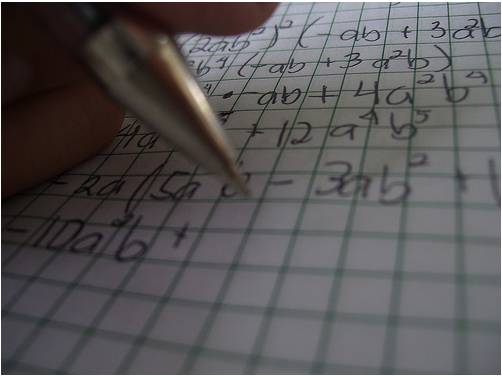Math 10 Honours Chapter 4:

•  LD

•  4.1
•  4.2
•  4.3

•  4.4
•  4.5

PPT

•  LD
•  SD

•  4.1
•  4.2
•  4.3

•  4.4
•  4.5

•  Rev.

Polynomial Functions

In this chapter, students will learn:

• to graph polynomial functions
• to factor and solve polynomial functions
Click for HomeworkMath 10 Honours Chapter 5:

•  5a
•  5.1
•  5.2
•  5.2b
•  5.3
•  5.4
•  5.5

PPT

•  5a
•  5.1
•  5.2
•  5.3
•  5.4
•  5.5
•  Rev.

Rational Functions

In this chapter, students will learn:

• to graph and solve rational functions
• to solve equations involving radicals, absolute values, and reciprocals
• to solve systems of equations and inequalities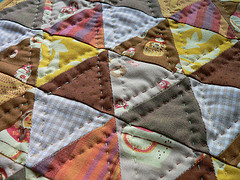Math 10 Honours Chapter 6:

•  6.1
•  6.2
•  6.3
•  6.4

•  6.4b
•  6R

PPT

•  6.1
•  6.2
•  6.3

•  6.4
•  6.4b

•  Rev.
Counting Principles
In this chapter, students will learn:

• to count using the Fundamental Counting Principles
• to count using Permutations and Combinations, and also understand what circumstances they are to be used
• to count when there are multiple cases
Click for Homework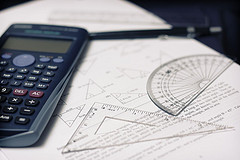Math 10 Honours Chapter 7:

•  7.1
•  7.2
•  7.3

•  7.4
•  7.5

•  7R
PPT

•  7a
•  7.1
•  7.2
•  7.3
•  7.4

•  7.5
•  Rev.
Probability

In this chapter, students will learn:

• to calculate probability for complex events
• to use tree diagrams and Venn diagrams to calculate probabilities
• to calculate probabilities with conditional events
• to calculate probabilities using Binomial distribution for events with only two outcomes
• Game: Deal or No Deal
Click for Homework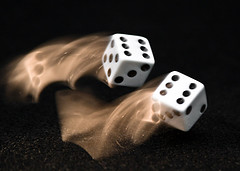Math 10 Honours Chapter 0:

•  Section 0.1 Introduction to Function Notations
•  Section 0.2 Introduction to Non-Linear Functions
•  Section 0.3 Introduction to Domain and Range
•  Section 0.3b Domain and Range for Composite Functions
•  Ch 0 Review

•  0.1
•  0.2
•  0.3
•  0.3b
•  0.R
Notes

•  0.1
•  0.2
•  0.3
•  0.R

Introduction to Functions

In this chapter, students will learn:

• to simplify basic function notations and composite functions
• to identify basic non-linear functions and the general formula
• to find the domain and range of basic functions graphically and algebraically